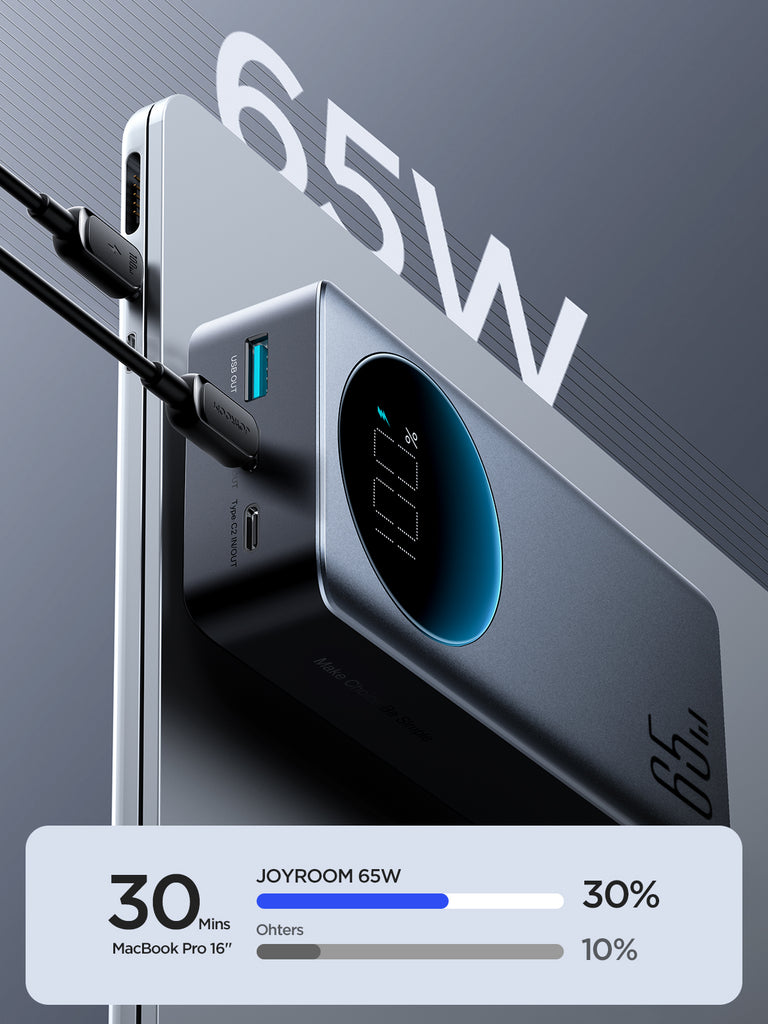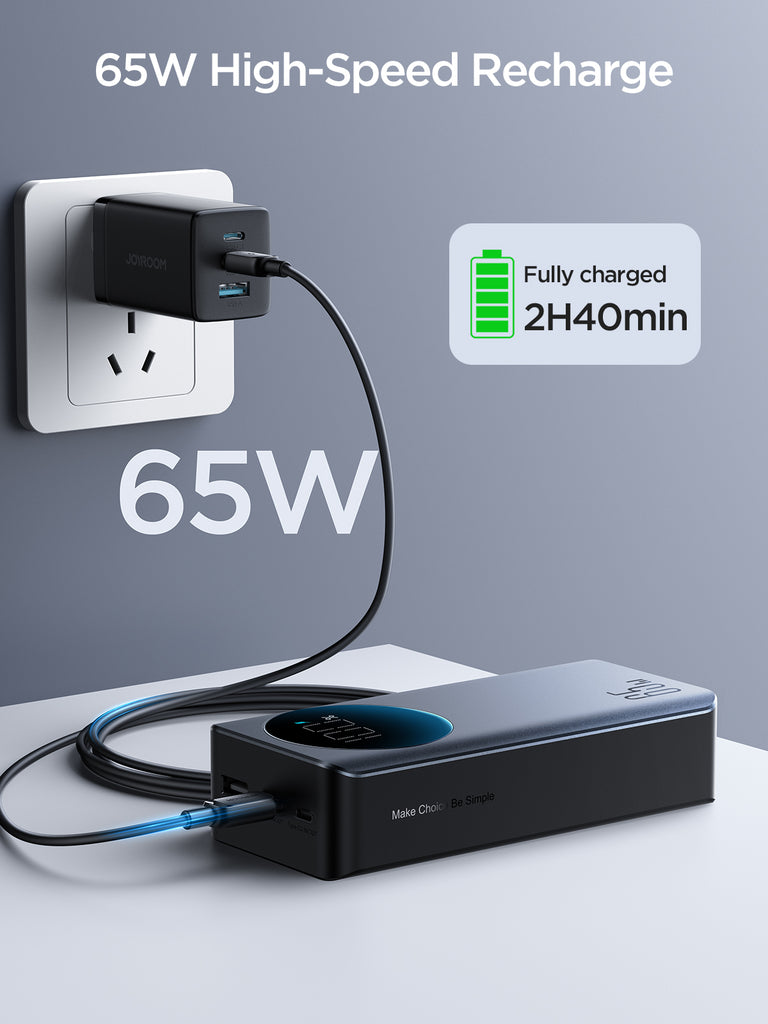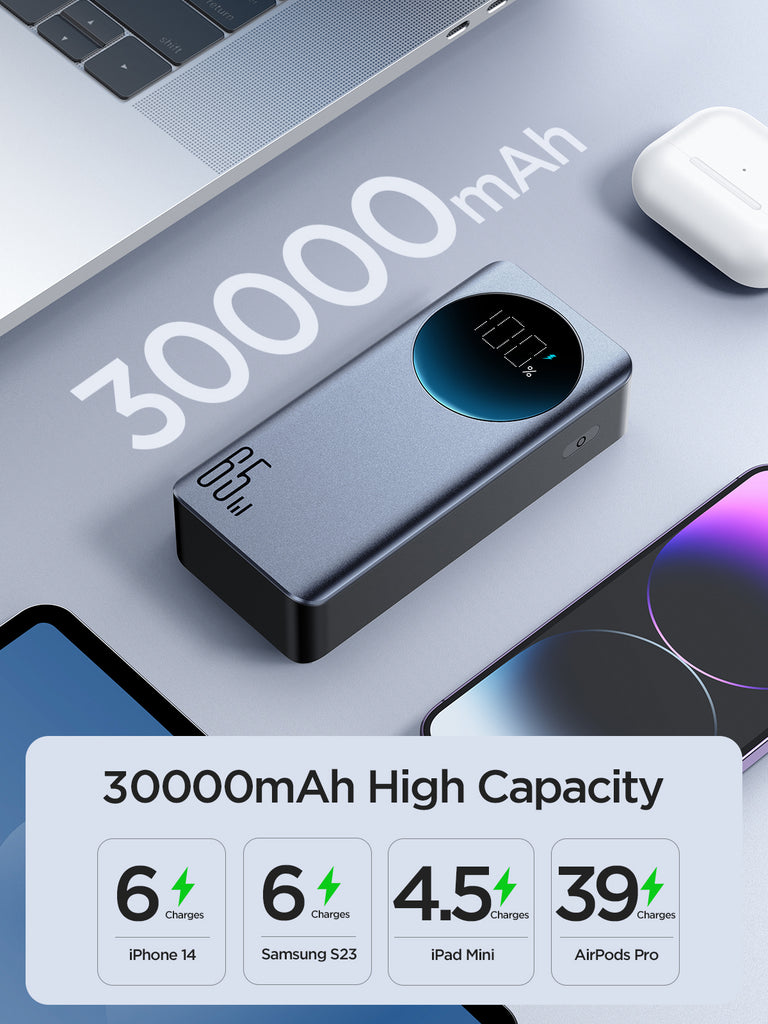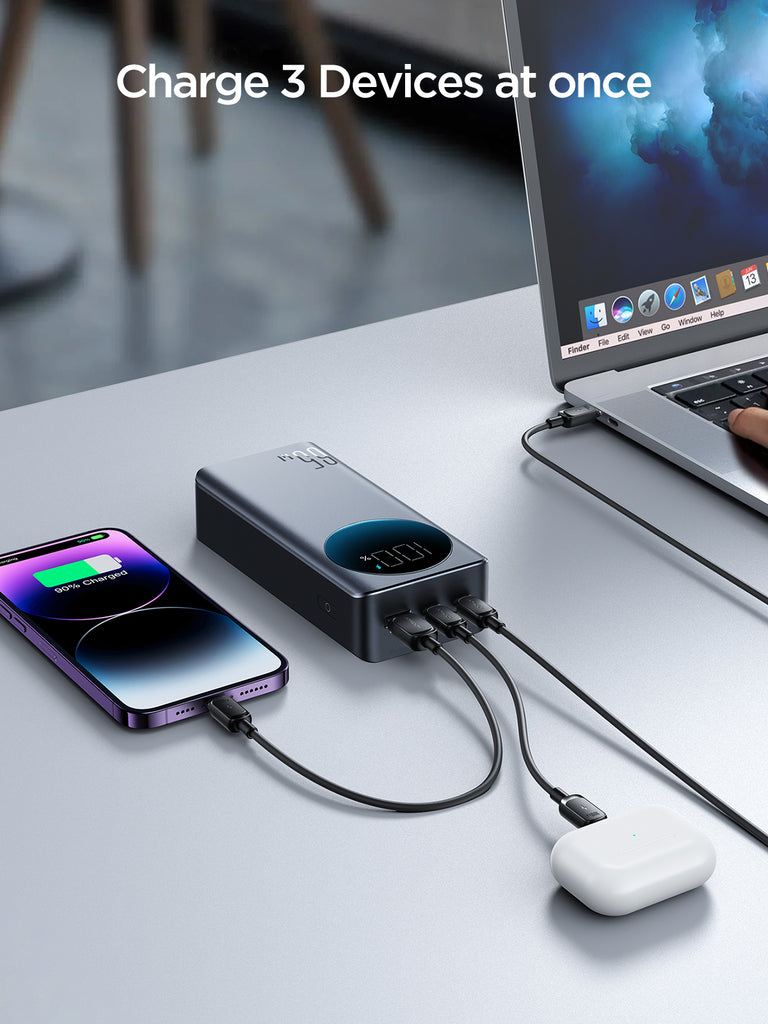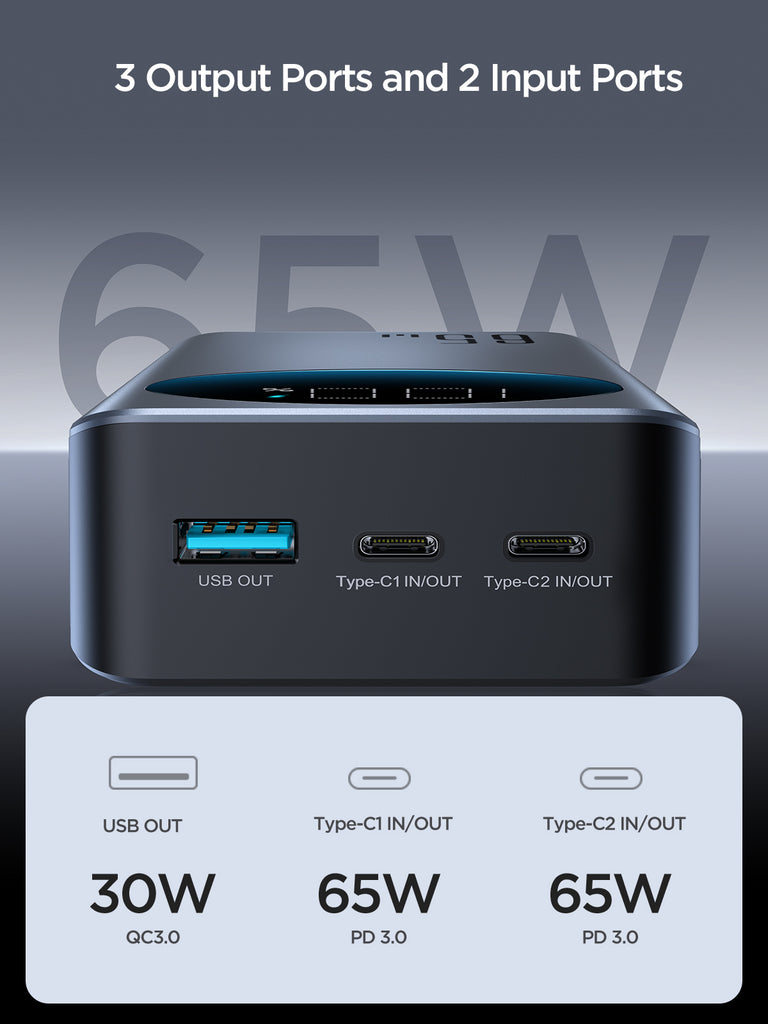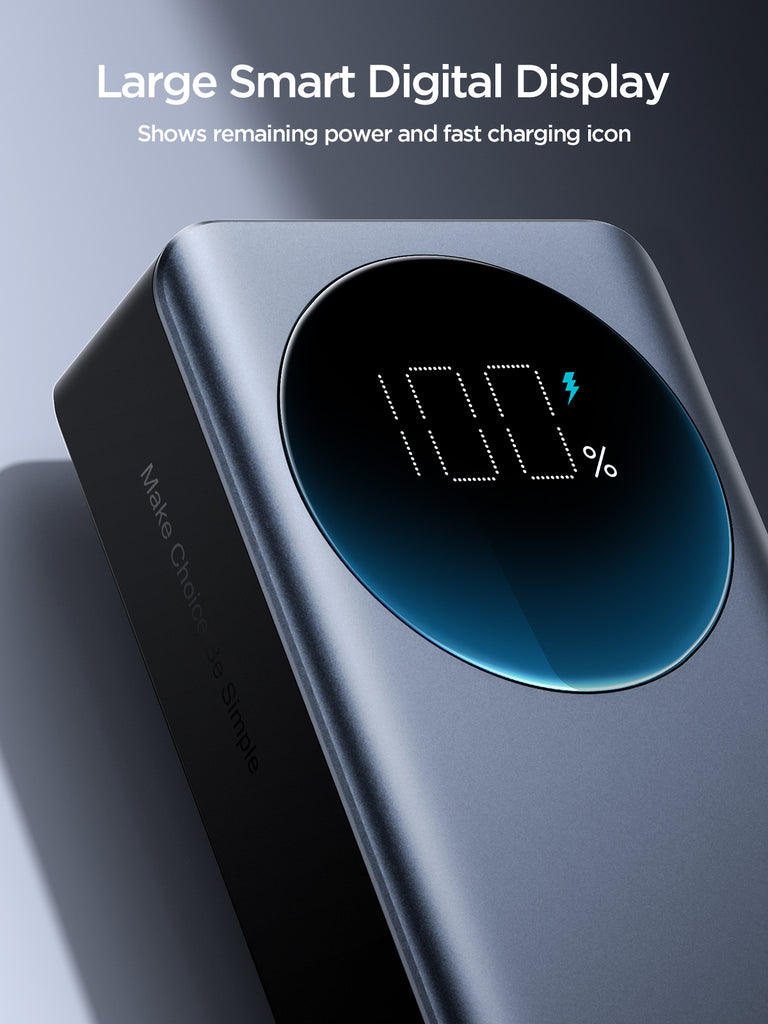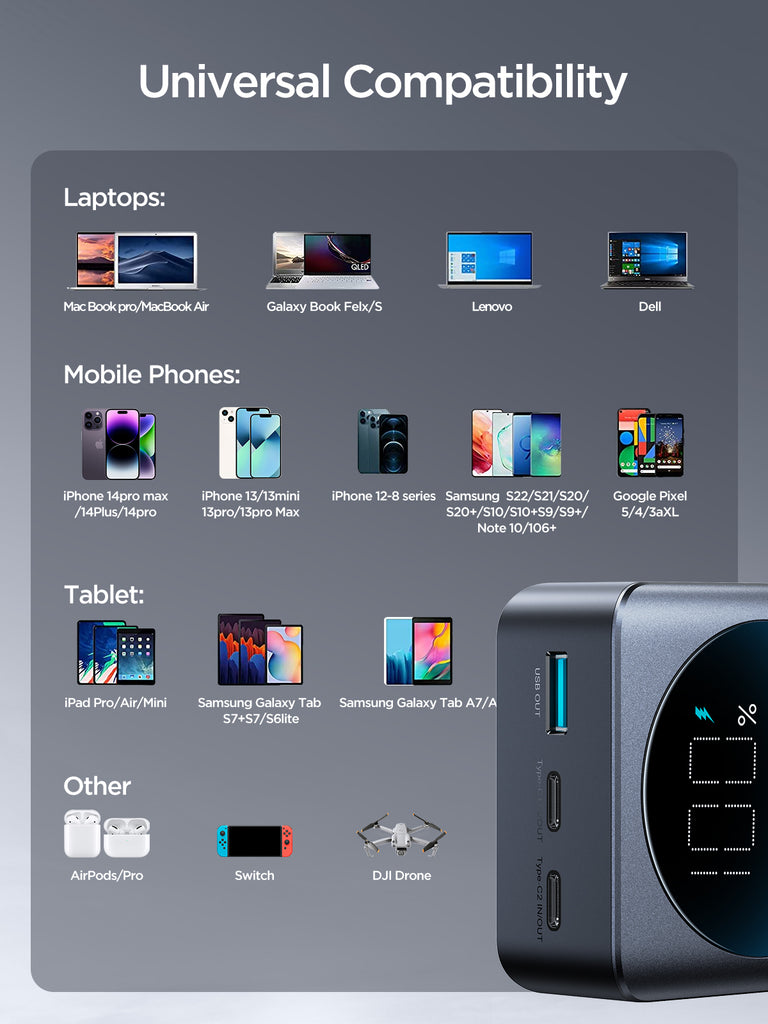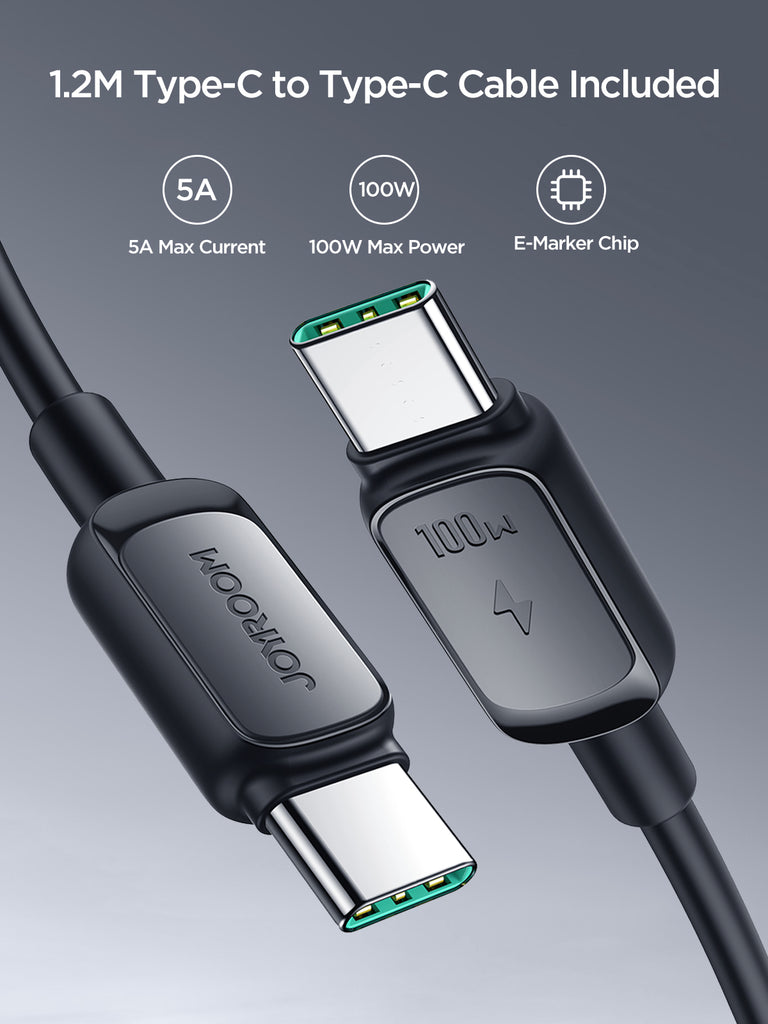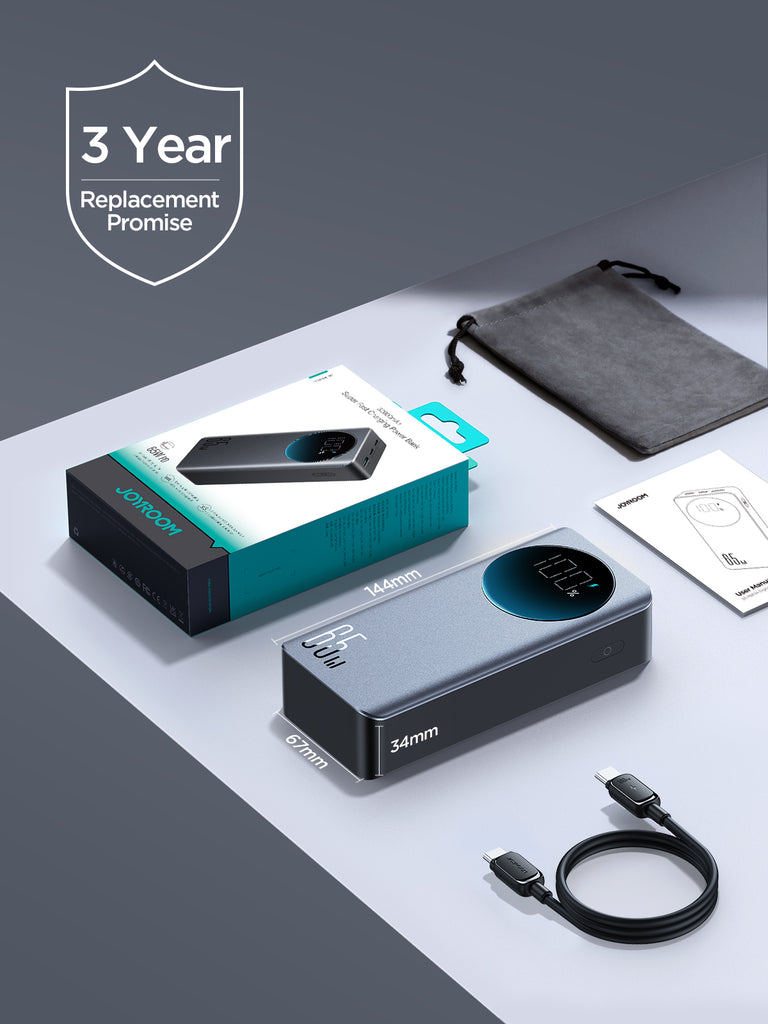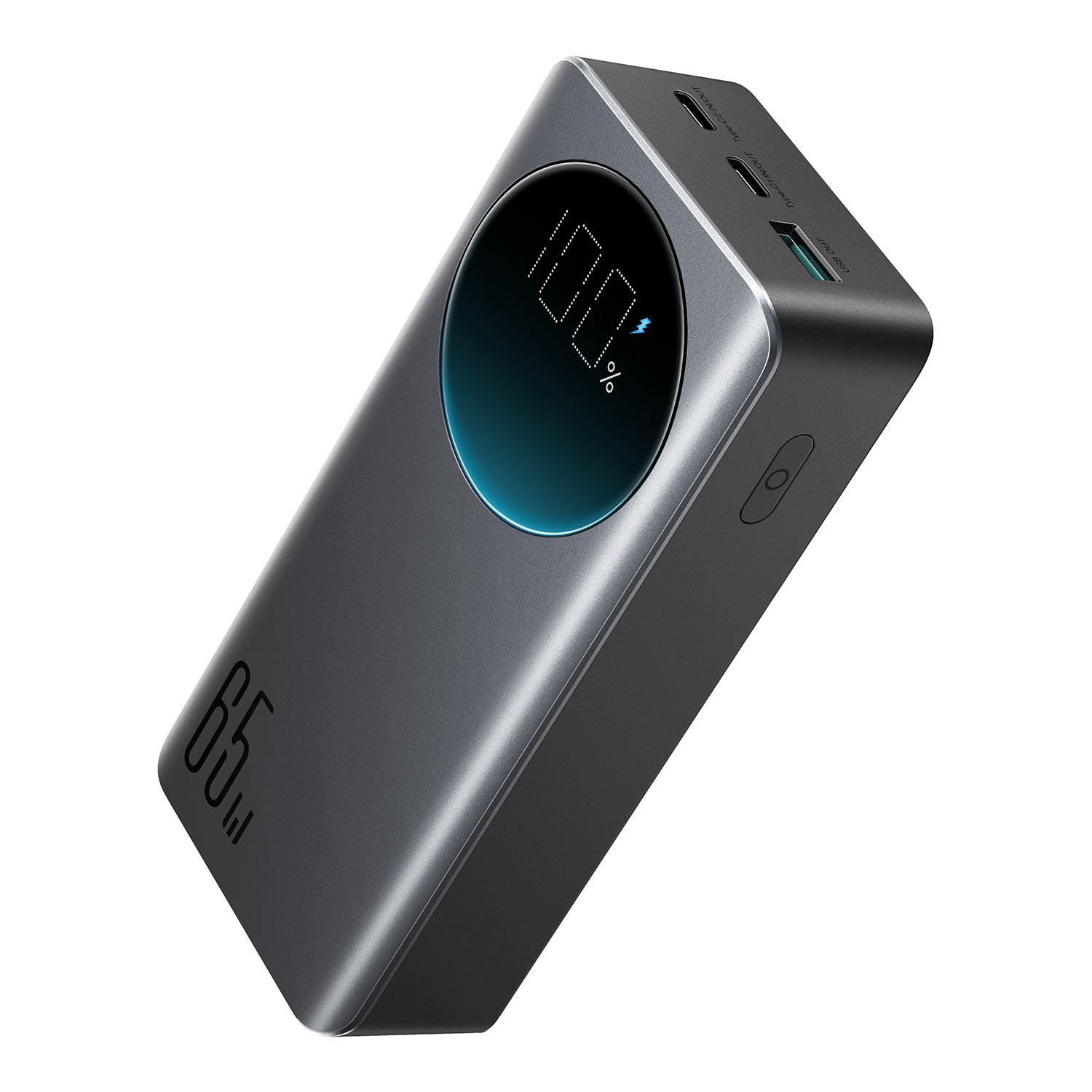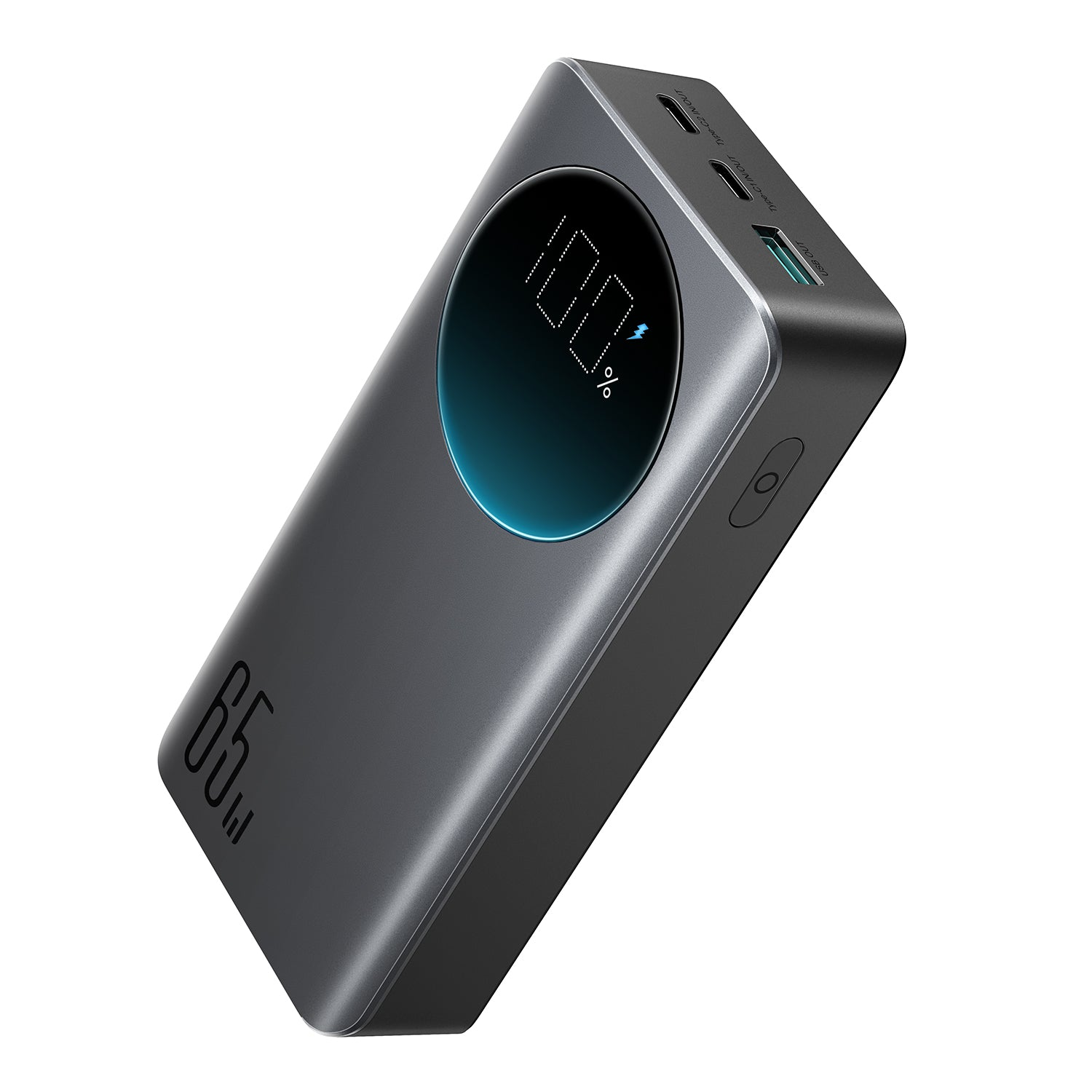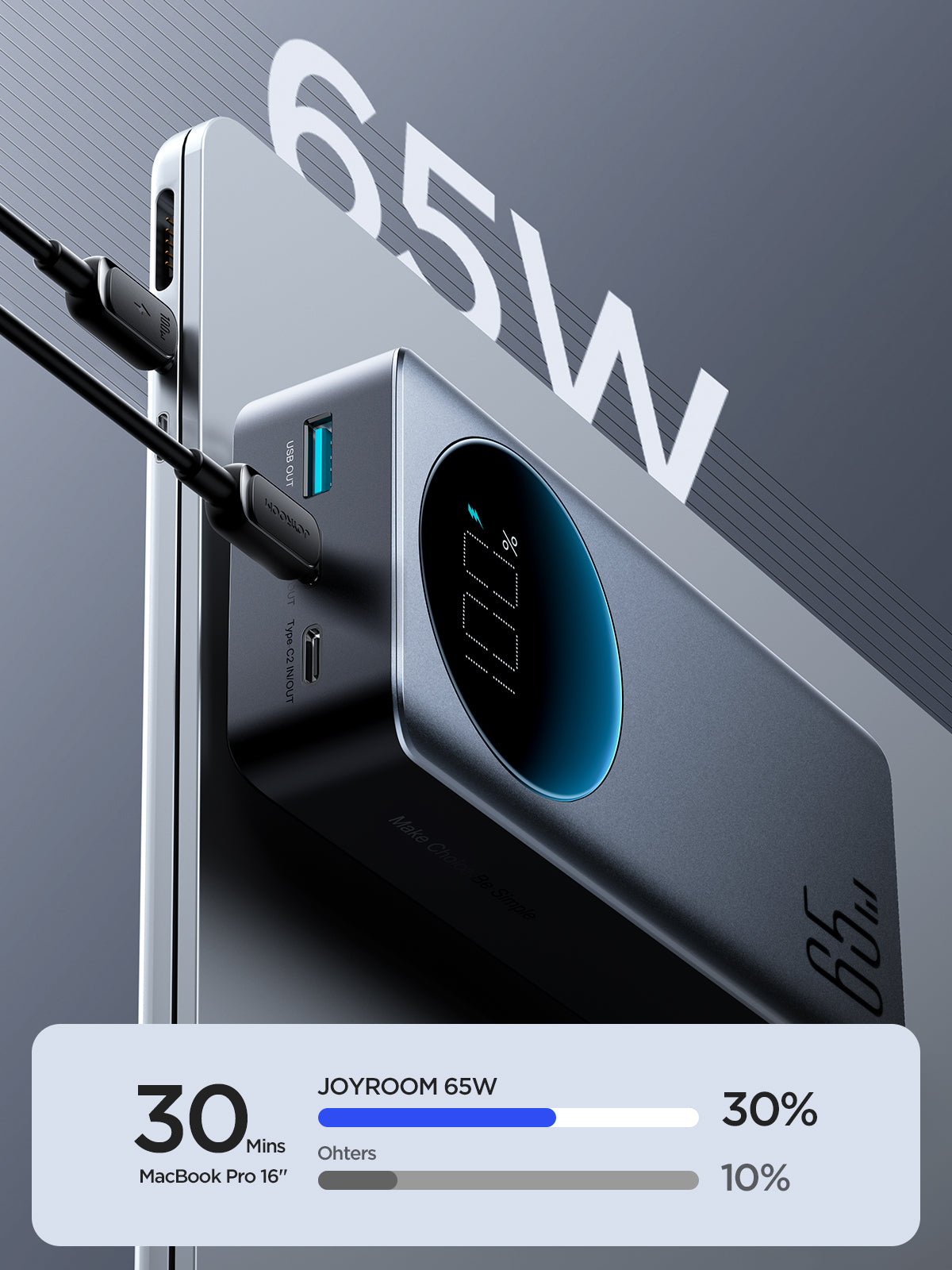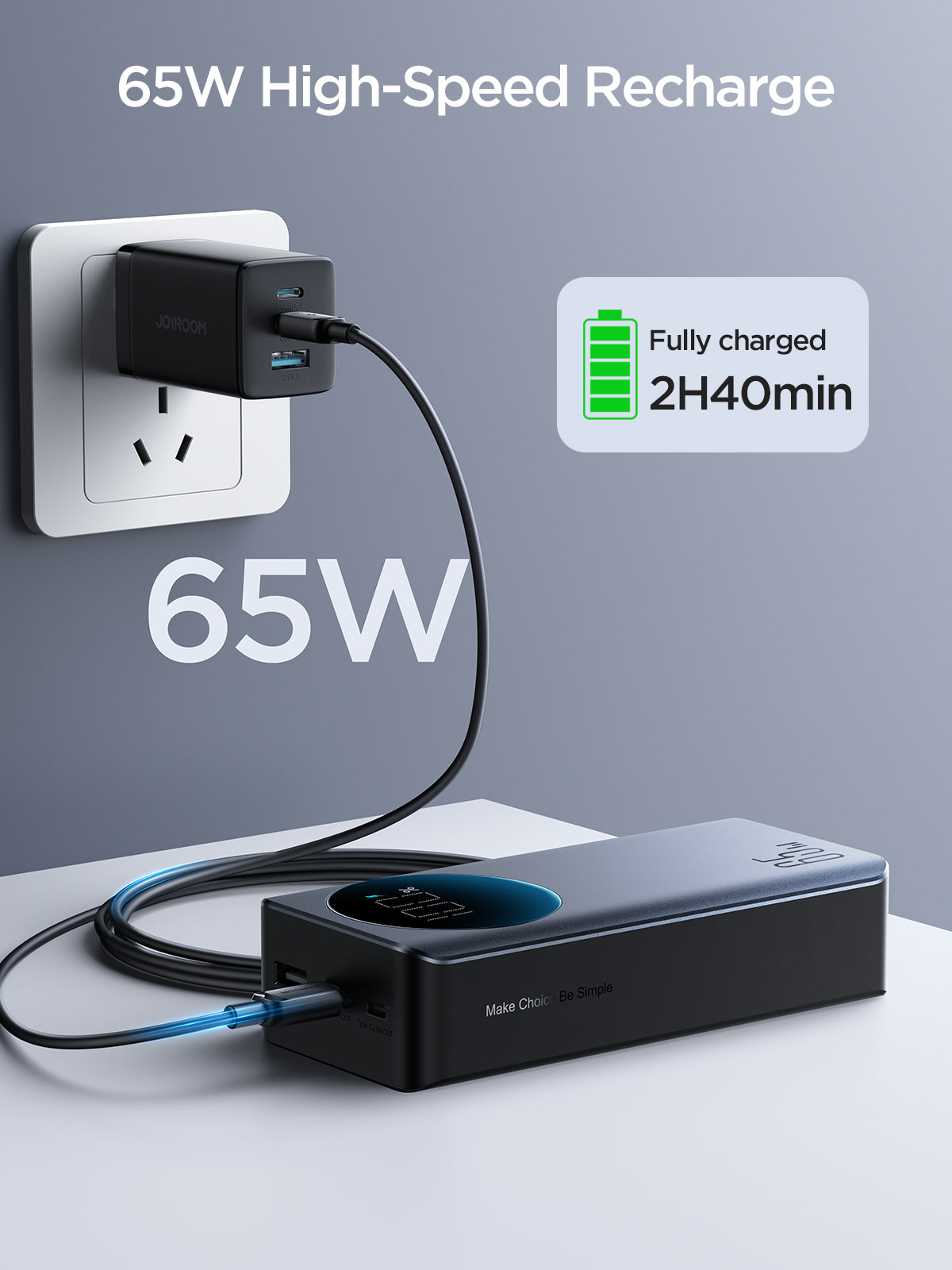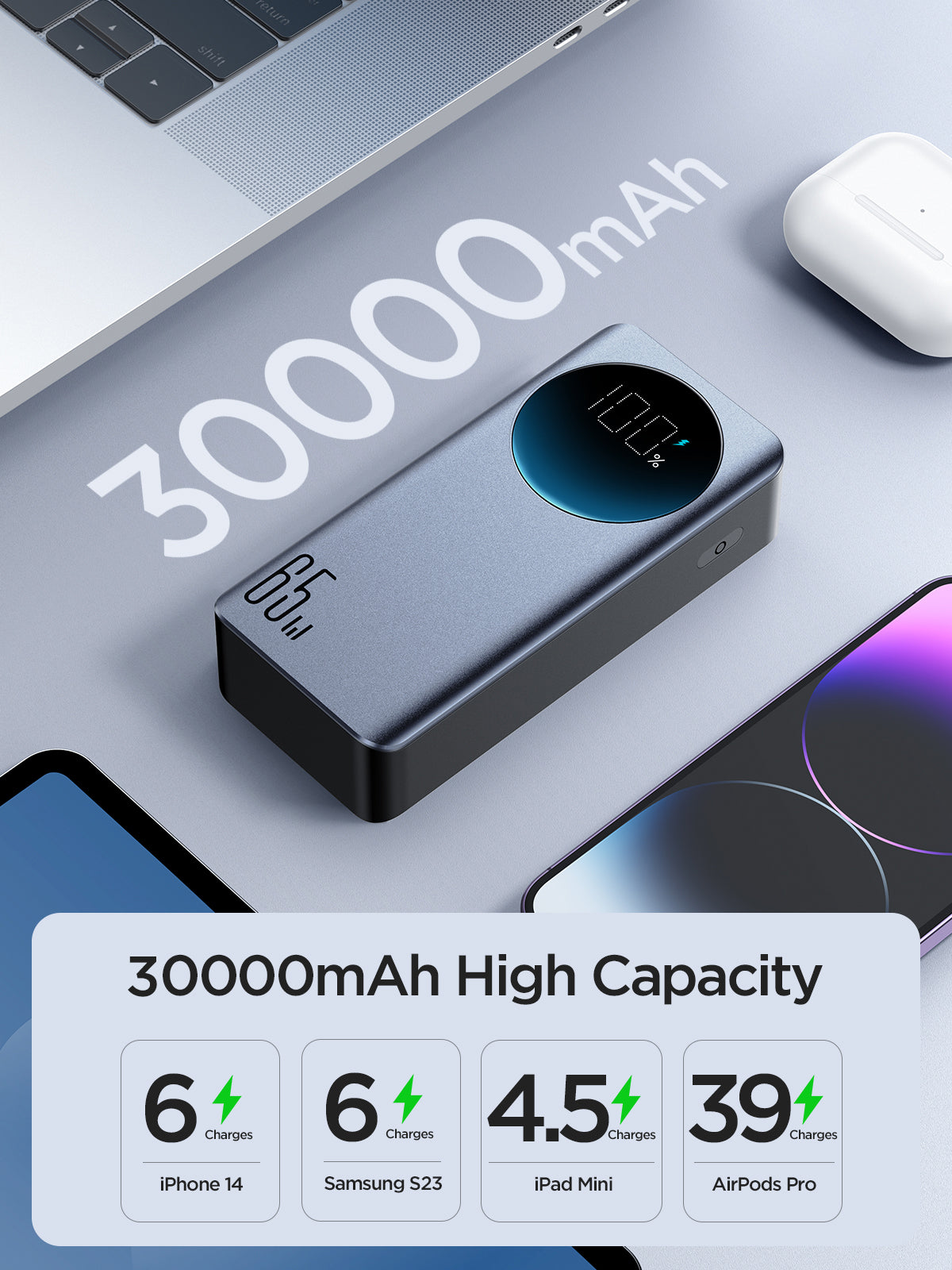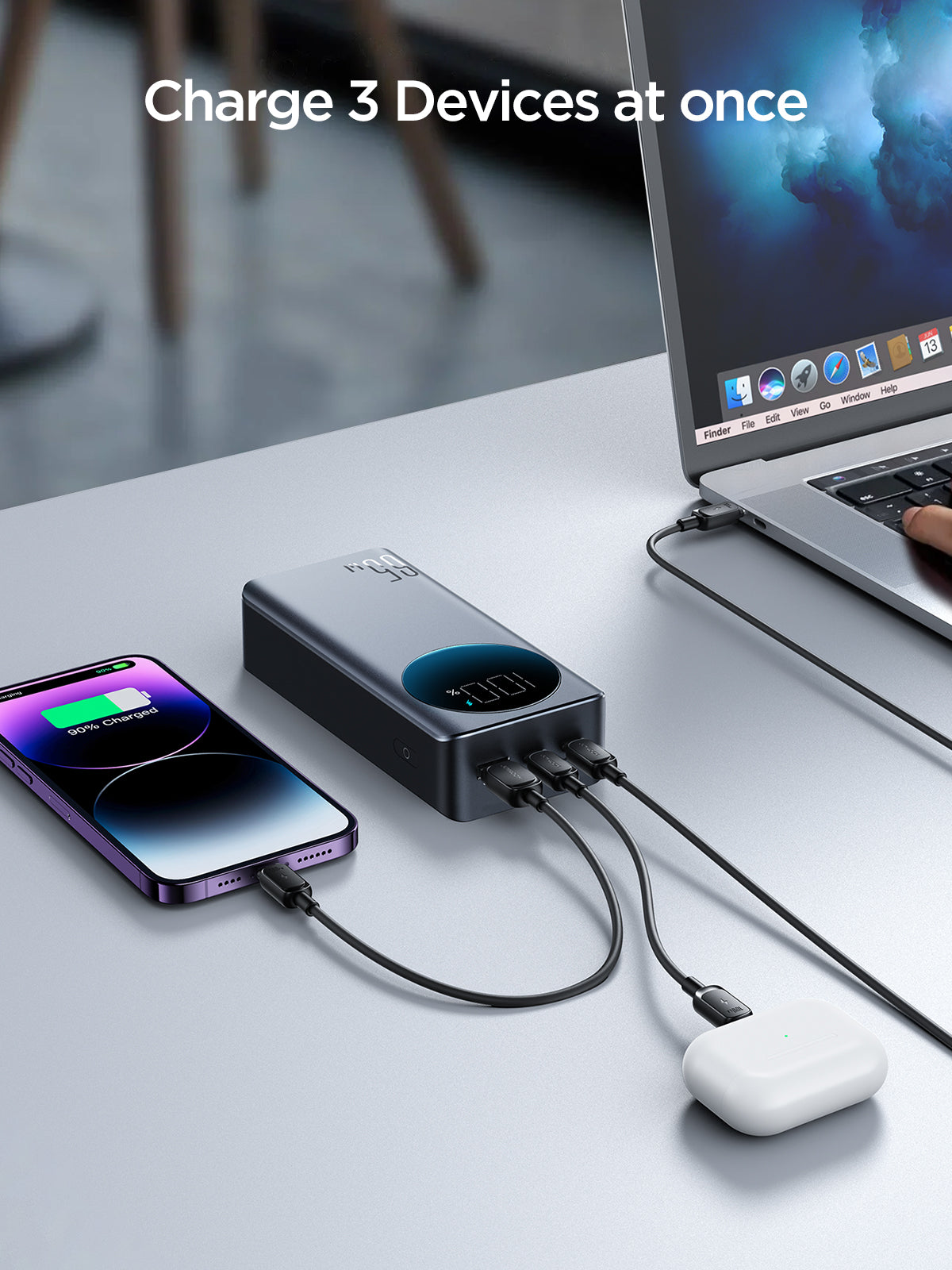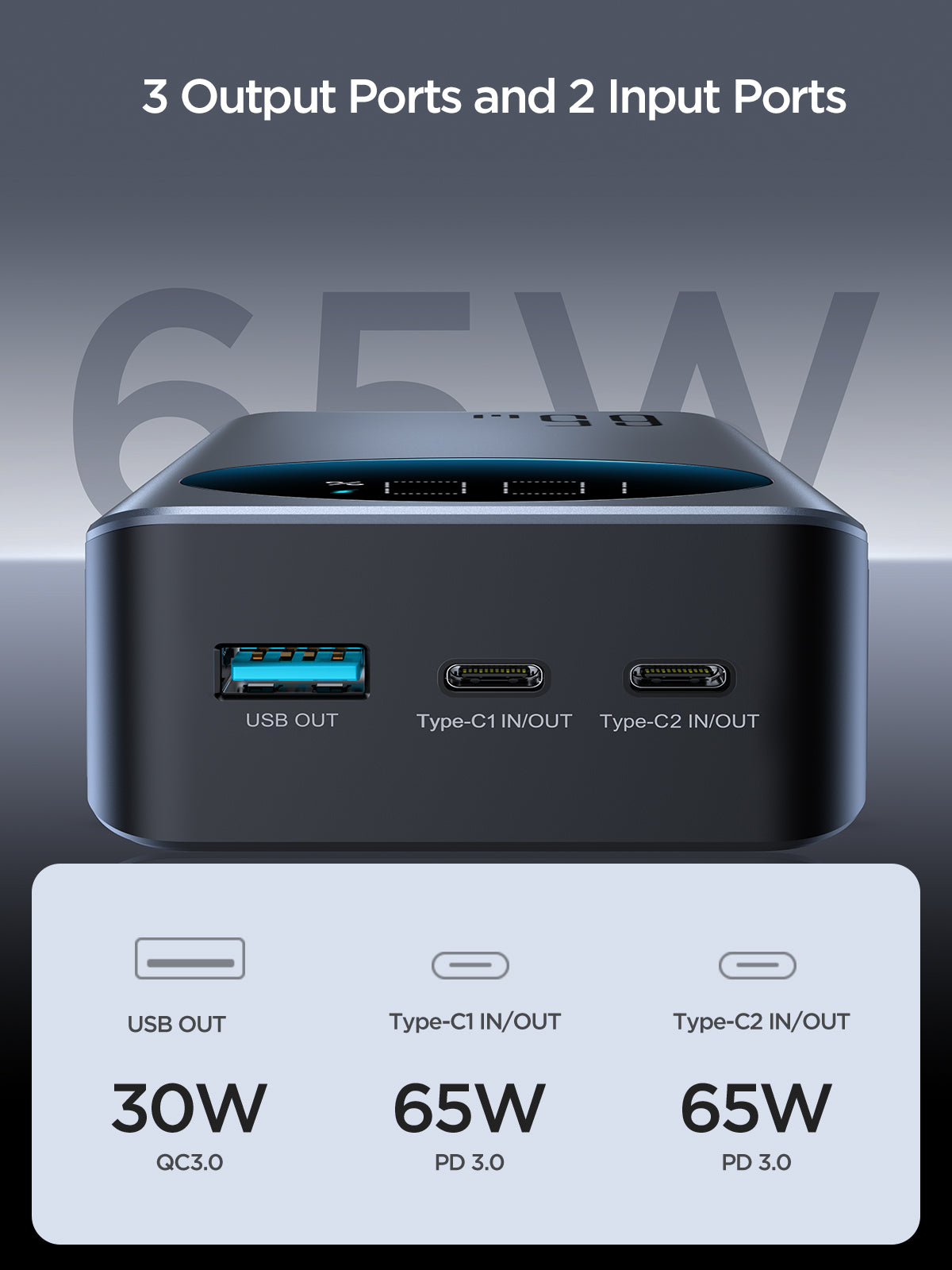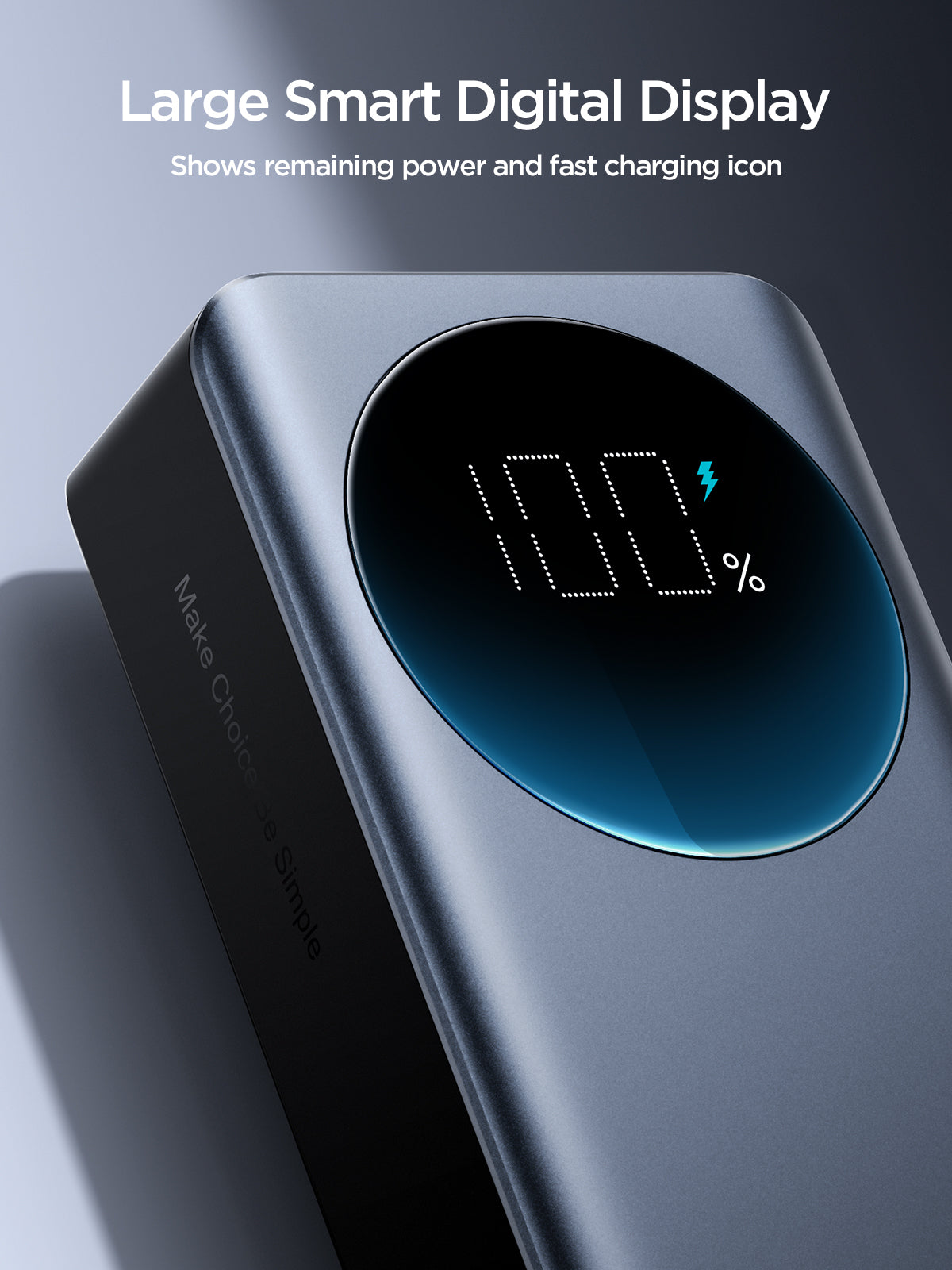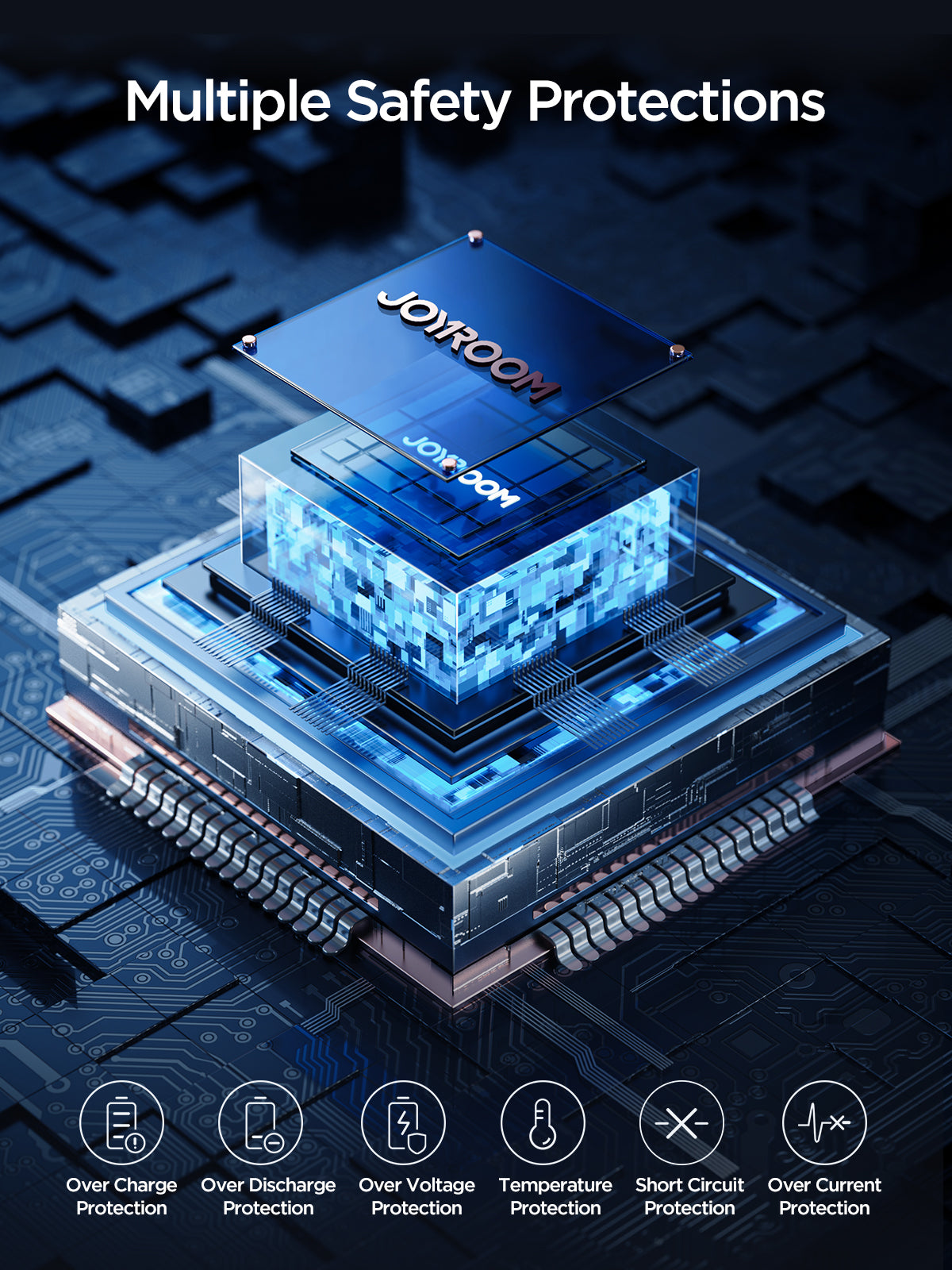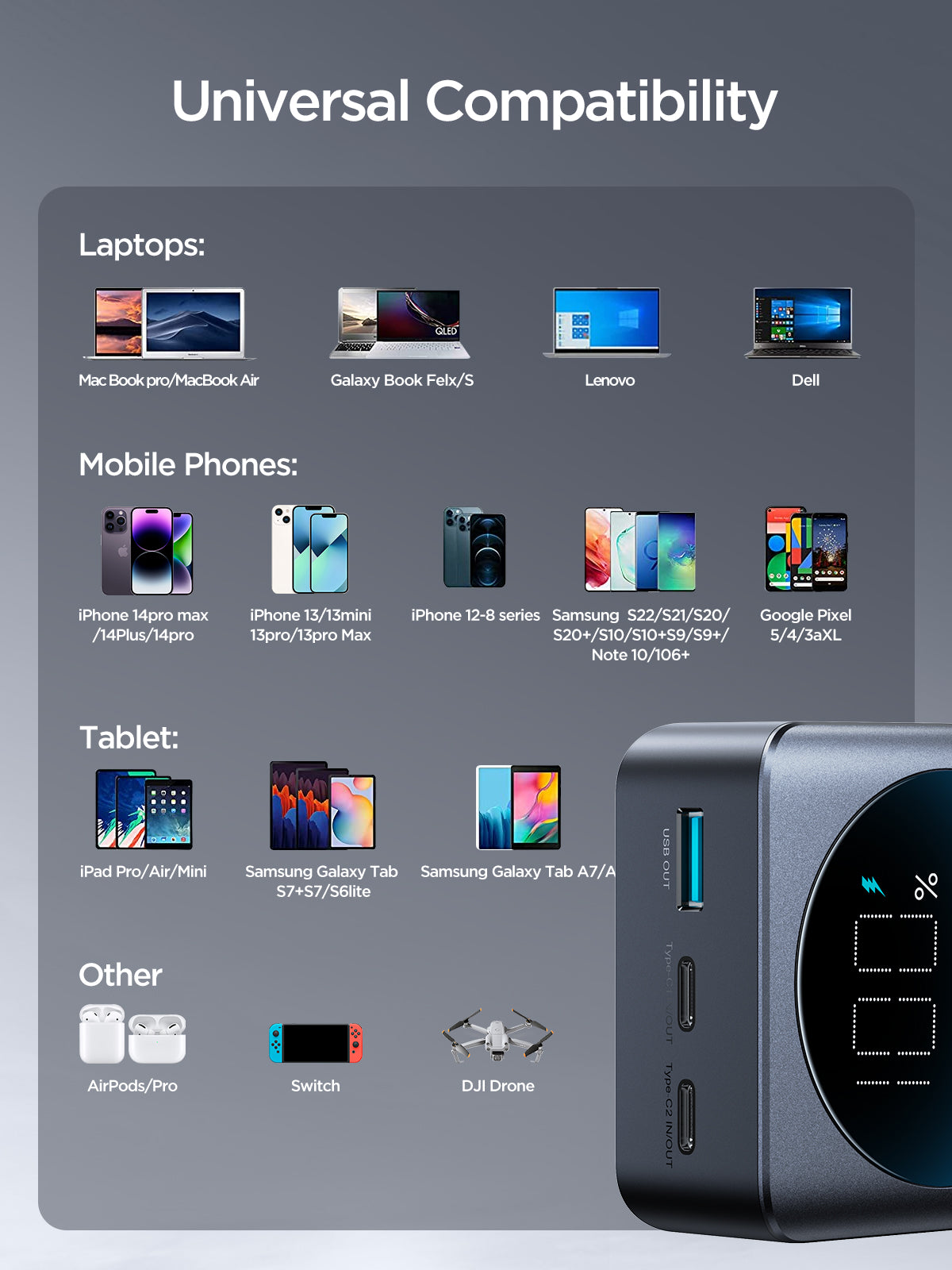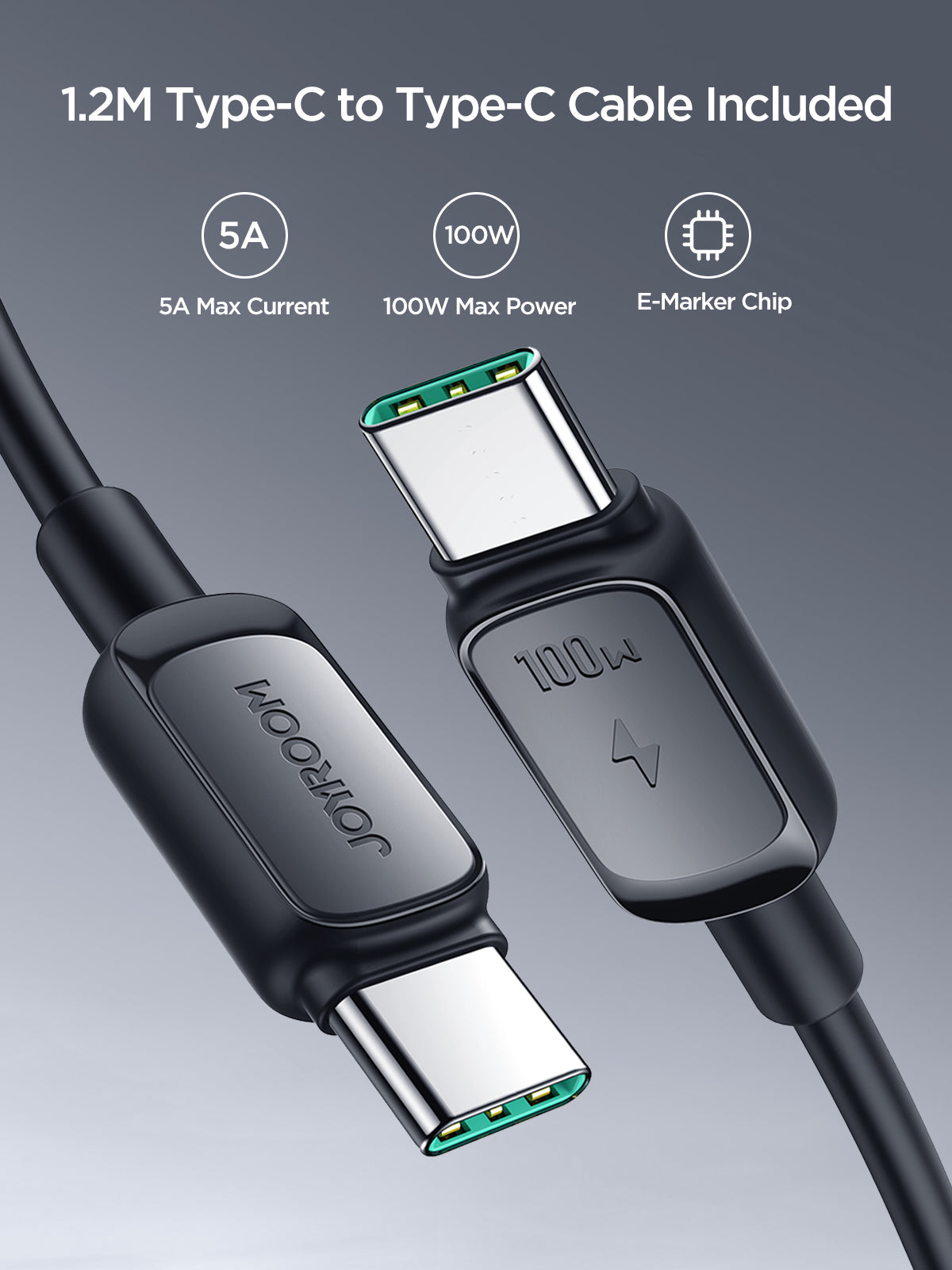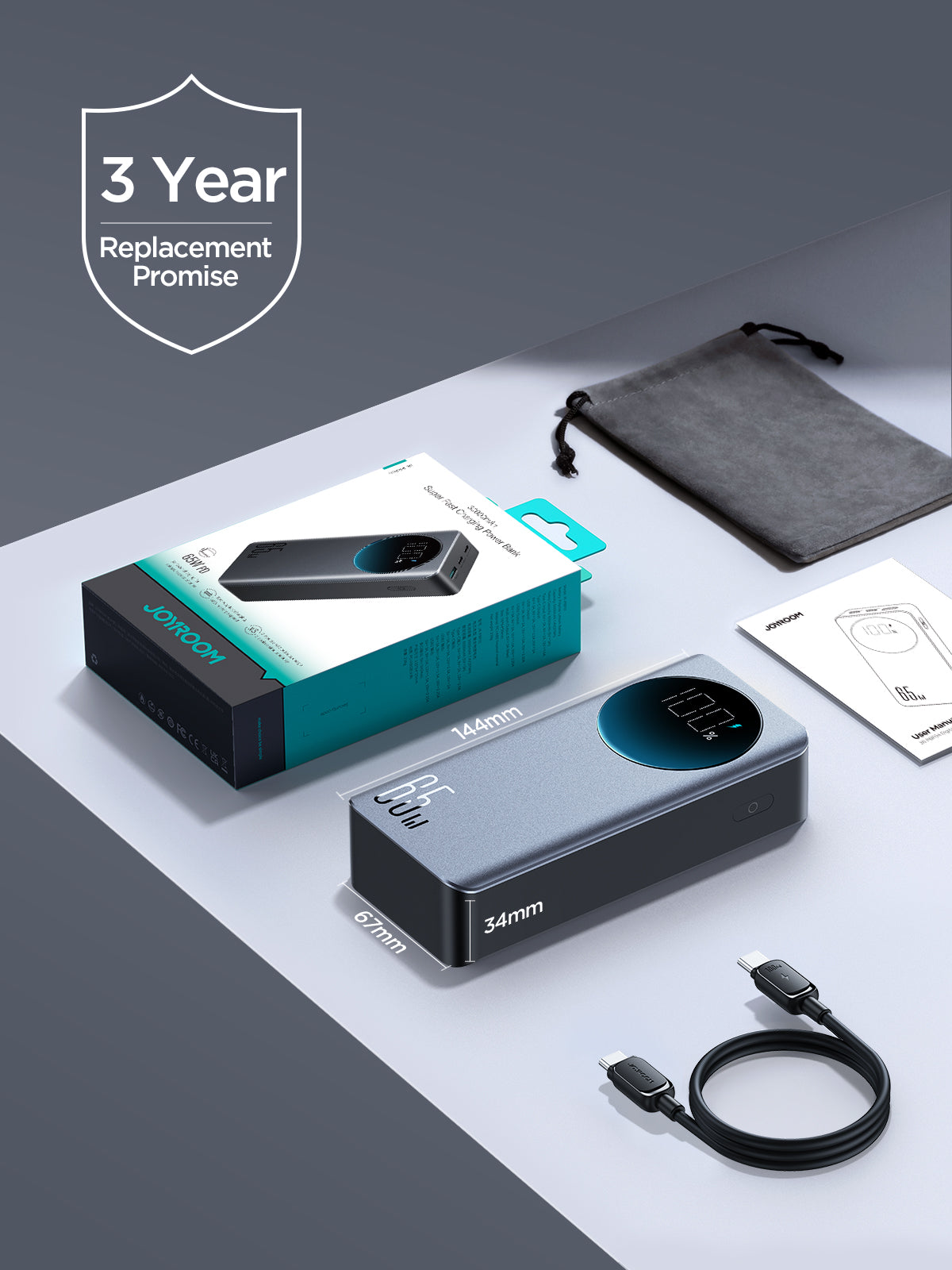# JR-PBF04/05 65W Digital Display Fast Charging Power Bank 20000mAh/30000mAh-Black (with Type-C To Type-C 100W 1.2m Cable-Black)

Sale price\$69.00
Color:Black
Capacity:20000mAh

# Specifications:

Model: JR-PBF04
Battery: Lithium Polymer Battery
Capacity: 20000mAh 3.7V(74Wh)
Rated Capacity: 12000mAh (5V=3A)
Type-C1 Input: 5V=3A,9V=3A,12V=3A,15V=3A,20V=3.25A
Type-C2 Input: 5V=3A,9V=3A,12V=3A,15V=3A,20V=3.25A
Type-C1 Output: 5V=3A,9V=3A,12V=3A,15V=3A,20V=3.25A
Type-C2 Output: 5V=3A,9V=3A,12V=3A,15V=3A,20V=3.25A
USB-A Output: 5V=4.5A,4.5V=5A,5V=3A,9V=3A,12V=2.5A
Multi-port Output: 5V=3A (Max)
Size: 144*69*29mm
Material: PC+ABS fireproof material
Net weight: 412g

Model: JR-PBF05
Battery: Lithium Polymer Battery
Capacity: 30000mAh 3.85V(115.5Wh)
Rated Capacity: 18000mAh（5V=3A）
Type-C1 Input: 5V=3A,9V=3A,12V=3A,15V=3A,20V=3.25A
Type-C2 Input: 5V=3A,9V=3A,12V=3A,15V=3A,20V=3.25A
Type-C1 Output: 5V=3A,9V=3A,12V=3A,15V=3A,20V=3.25A
Type-C2 Output: 5V=3A,9V=3A,12V=3A,15V=3A,20V=3.25A
USB-A Output: 5V=4.5A,4.5V=5A,5V=3A,9V=3A,12V=2.5A
Multi-port Output: 5V=3A (Max)
Size: 144*67*34mm
Material: PC+ABS fireproof material
Net weight: 495g

# Features:

1. 65W output, 6X faster charging for laptops, tablets, phones
2. Two-way fast charging, 65W recharge speed
3. 2 inputs and 3 outputs,charge 3 devices simultaneously
4. JR-PBF04: 20000mAh large capacity, 5-day battery life, perfect for travel; JR-PBF05: 30000mAh large capacity, 7-day battery life, perfect for travel
5. Large smart digital display, precisely indicates battery level & fast charging icon
6. Smart temperature control chip,safe charging with less heat
7. Package contents: power bank, travel pouch, 100W C T C charging cable 1.2m, User Manual
8. Supports PD3.0, QC3.0, Samsung PPS/AFC, SCP/FCP fast charging protocols, etc.